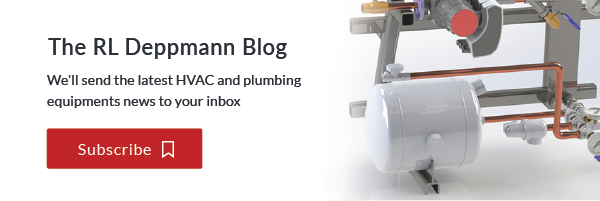# Expansion and Compression Tanks in Hydronic Systems (Part 4): Acceptance Volume

Norm Hall
/
December 24, 2018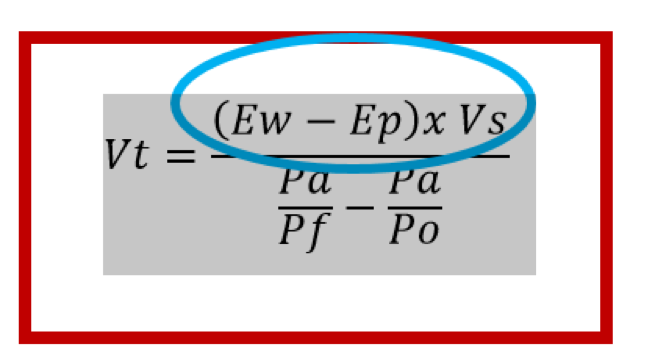Acceptance volume should be one of the values shown in the hydronic system tank schedule. In the last two (Part 2 and Part 3) R. L. Deppmann Monday Morning Minutes, we addressed the pressures required in the denominator of the tank formula. Today, we examine the temperatures and fluid type which are required inputs for selection programs to determine the acceptance volume of the tank.

We are examining this subject in detail in this R. L. Deppmann Monday Morning Minutes, but are also offering a shorter video version from one of our sales engineers, Mark Fine.

## What is Acceptance Volume?

The numerator of the formula above is called the acceptance volume. In the formula (Ew– Ep) * Vs, (Ew– Ep) is the expansion of the fluid minus the expansion of the pipe and Vs is the system volume in gallons. This is the volume required as the fluid is heated without concern for pressure.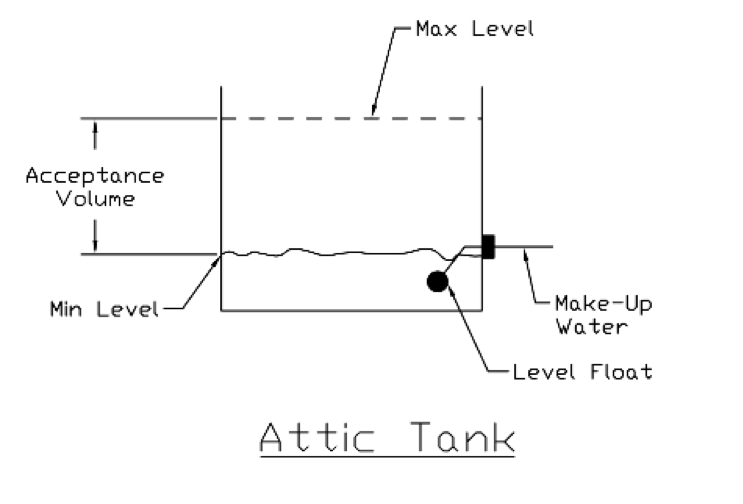Back when the original gravity flow hydronic systems were designed, there was an open expansion tank normally located in the attic. As the boiler fired, the water would expand and the level in the tank would rise. The starting pressure was atmospheric and so was the ending pressure since the tank was open. So the tank volume equaled the acceptance volume. As the water in the system was heated, it would expand into the open expansion tank. The tank had to have enough volume to handle the increase from the starting temperature to the maximum system temperature.

Today, it is rare to use an open expansion tank. Now we use compression or expansion tanks that can handle the acceptance volume while keeping the pressure between the fill pressure and the maximum pressure.

## What is the Fill Temperature and Maximum Temperature?

Two inputs required in the B&G ESP Systemwize tank selection program are the fill temperature and the maximum temperature. We are looking for the expansion of the fluid in the system from when it is filled to the maximum temperature in the system.

The fill temperature is normally defaulted to 40°F in our area here in the north. The assumption at the time of design is that we don’t know if it will be filled in the winter or the summer so we default to the lowest number. You can change it to the ambient temperature of the water in your area. This number is NOT the return system temperature. It is the fill temperature from the municipality or well.

The maximum temperature used will vary depending on your comfort level of the system operation. By the book, this is the average temperature of the entire system volume. For example, assume that the system is a heating system with a design supply temperature of 180°F and a 140°F return temperature. This is the example we use in the Part 1 Quiz. We can assume that in the dead of winter half of the system water or the supply is at 180°F while the other half or the return is at 140°F. The average maximum temperature is 160°F.

What happens in the spring and fall when the system does not require as much heat? If we assume there is a reset schedule, then the average temperature will be lower so the maximum remains 160°F. But, if there is no reset schedule or it is not working as designed, then when we send out 180°F fluid in the spring or fall, the return temperature will be much higher than 160°F. To be safe we may use 180°F as the maximum temperature which will result in more safety factor to the selection.

## Why Do We Need the Fluid Type for Expansion Tank Size Selection?

The expansion of water from one temperature to another is different than glycols or other fluids. The expansion is calculated for you in the B&G Systemwize program. This calculation is not a mystery.

EF = [((specific volume at hot Temp.)/ (specific volume at cold Temp.))-1]

The calculation of the required tank acceptance volume is (Ef – Ep) * system volume. Let’s look at an example and assume the system has 50% ethylene glycol but you did not change the default from water. Assume you have a closed hydronic heating system with a maximum temperature of 180°F and a 40°F ΔT with reset. Assume the system volume is 1400 gallons. Let’s also assume a starting pressure of 12 PSIG and a maximum system pressure of 27 PSIG. What happens to the acceptance volume?

The acceptance volume for water is 29.29 gallons and the acceptance volume for glycol is 52.64 gallons. You will hear about this error since the pressure relief valve will start to weep at 115°F average temperature as a result of the incorrect fluid properties being used.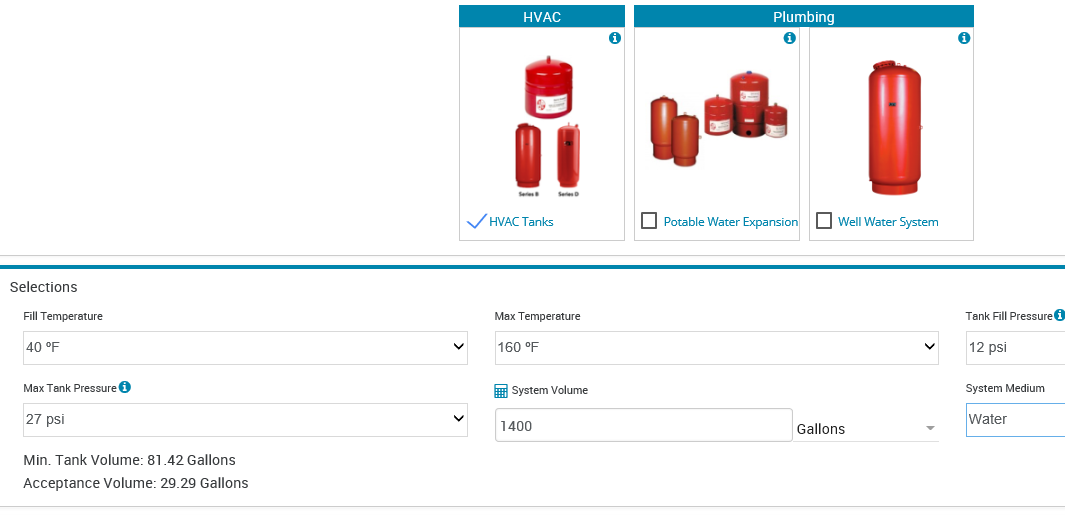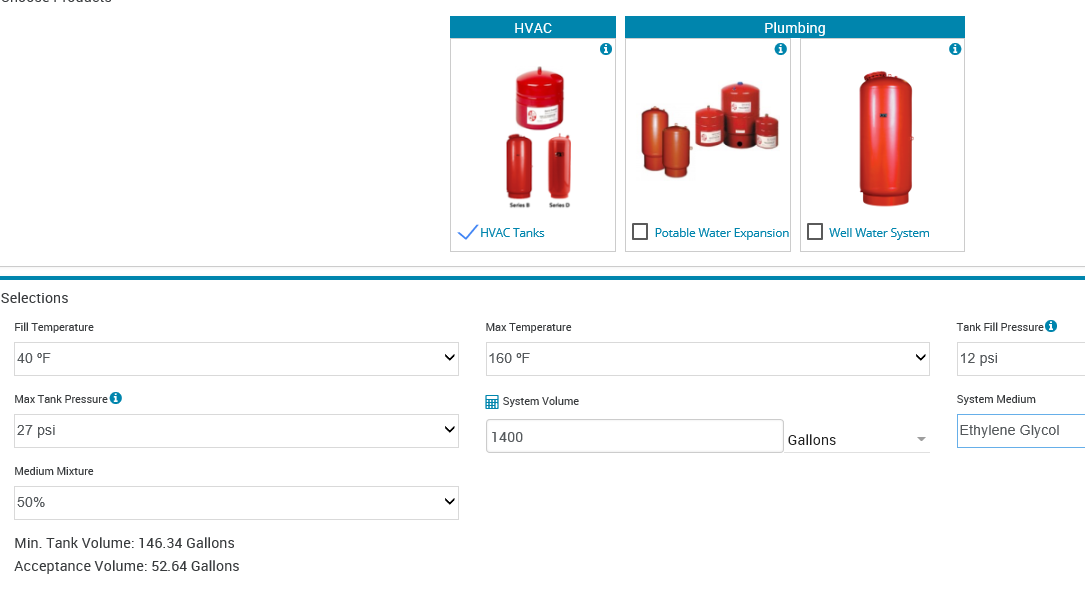Now that you know how to determine the acceptance volume, the next R. L. Deppmann Monday Morning Minutes will look at the “old style” compression tanks and air control systems.

### Check out the rest of the Expansion and Compression Tanks in Hydronic Systems Series: